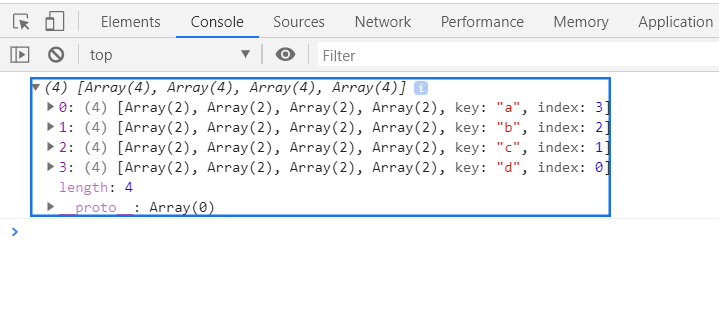Open in App
Not now

# D3.js stackOrderReverse() Method

• Last Updated : 15 Sep, 2020

The d3.stackOrderReverse() method orders the series based on the ordering of the keys as defined by stack.keys() method.

Syntax:

```d3.stackOrderReverse(series)
```

Parameters: This function accepts a single parameter as mentioned above and described below:

• series: This is the series based on the ordering of the keys to be ordered.

Return Value: This method returns no value.

Example: Ordering the stack using d3.stackOrderReverse function.

## HTML

 ```<``html``>``<``head``>``    ``<``meta` `charset``=``"utf-8"``>`` ` `    ``<``script` `src``=``        ``"https://d3js.org/d3.v5.min.js"``>``    `````` ` `<``body``>``    ``<``h1` `style``=``"text-align: center; color: green;"``>``        ``GeeksforGeeks``    ```` ` `    ``<``center``>``        ``<``canvas` `id``=``"gfg"` `width``=``"200"` `height``=``"200"``>``        ````    ```` ` `    ``<``script``>``        ``var data = [``              ``{letter: {a: 3840, b: 1920, c: 960, d: 400}},``              ``{letter: {a: 1600, b: 1440, c: 960, d: 400}},``              ``{letter: {a:  640, b:  960, c: 640, d: 400}},``              ``{letter: {a:  320, b:  480, c: 640, d: 400}}``            ``];`` ` `        ``var stackGen = d3.stack()``            ``// Defining keys``            ``.keys(["a", "b", "c", "d"])``            ``// Ordering``            ``.order(d3.stackOrderReverse);``       ` `        ``var stack = stackGen(data);``         ` `        ``console.log(stack);`` ` `    `````` ` ``

Output:My Personal Notes arrow_drop_up